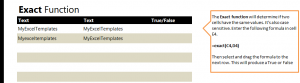# Exact Match Function

17
AprLearn to use the exact match function in Excel spreadsheets to find if two entries are exactly the same or not. This formula is easy to use and requires only a simple formula. This particular function is used when you are looking for duplicate content, comparing answers to a master sheet, or finding errors in entries.

The downloaded example spreadsheet contains two populated rows, one with an exact match and one without. Exact match is case sensitive and counts spaces and symbols.

Click cell E4 and enter the formula:

=exact(C4,D4)

This tells Excel to compare cells C4 and D4. The answer returned should be True.

Now go to cell E5 and enter:

=exact(C5,D5)

You will get the answer of False, as the capitalization is inconsistent.

To avoid entering in the formula each time for every row you are working with, drag the corner of cell E4 (or your starting cell) down the column you want the answers to appear. Excel will automatically return the results to you and apply the formula to all of the cells in that selected column.